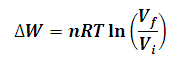# Work Done in Isothermal Process Calculator

This Calctown Calculator calculates the work done by the system in an Isothermal process.

mol
K
m3
m3

#### Result

Jwhere

ΔW = work done

n = number of moles

R = universal gas constant

T = temperature

Vi = initial volume

Vf = final volume